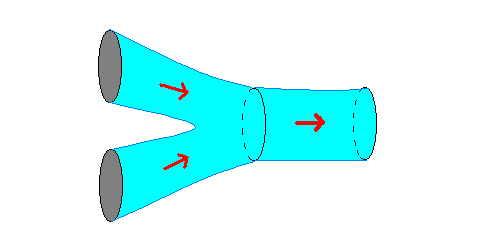## Wednesday, September 16, 2020 ...//### A fun trinification $$E_8$$ string-inspired model

This funny yet silly 2011 song about Greece's living beyond its means (and how it should be inspired by some Czech tricks; the song targets the Czech character more than the Greek one) has collected almost half a million views. Slovaks recorded a similar song about the Greek debt (secured by Russia), a parody on Modus' Little Pieces of Glass (1984).

Two months ago, I discussed a Mexican string-inspired $$E_8$$-based model of particle physics. "Not quite stringy" but "suspiciously string-like" models with $$E_8$$ in ten dimensions must be getting more popular. Today, a Athens-Munich-Potsdam-Geneva paper

$$N=1$$ trinification from dimensional reduction of $$\NNN=1$$, 10D $$E_8$$ over $$SU(3)/U(1)\times U(1)\times\ZZ_3$$ and its phenomenological consequences
by Manolakos, Patellis, and Zoupanos uses a compactification of the $$E_8$$ gauge theory on a 6-dimensional manifold with some Wilson lines, too. But while the Mexican paper chose an orbifold of the (flat) six-torus, this Greek paper exploits a curved, fancier manifold, namely$\ZZ_3 \backslash SU(3) / (U(1) \times U(1)).$ At least I hope that the coset written on both sides is correct, they were a bit sloppy about it. The Wilson lines chosen on the $$\ZZ_3$$ transformations break $$E_8$$ to $$SU(3)^3$$, the gauge group of "trinification" models (a relative of "unification" models that is, in some sense, equally "unified" as unification, assuming a generalized usage of "unified").

The number "three" seems to be everywhere. It's helpful that the group $$SU(3)$$ has the center isomorphic to $$\ZZ_3$$; in the usual representations using the $$3\times 3$$ unitary matrices, the center are the unit matrices multiplied by a cubic root of unity. Now, locally, the manifold is $$SU(3) / U(1) \times U(1)$$ which is not Ricci-flat and that is why it doesn't sound "great as a string theory" right away but it has a related, somewhat weaker, string trait.

In July 2001, the late Michael Atiyah and Edward Witten wrote a beautiful geometric paper on M-theory,
M-Theory Dynamics On A Manifold Of $$G_2$$ Holonomy
They studied the spectrum and interactions of M-theory (11-dimensional theory) on manifolds which are 7-dimensional cones; the whole cones end up having the exceptional $$G_2$$ holonomy. The base of the cone could have been one of the three options: $$\mathbb{C}\mathbb{P}^3$$, $$S^3\times S^3$$, and... yes, exactly our Greek manifold $$SU(3) / U(1) \times U(1)$$. All these three manifolds have the number "three" deeply embedded in them.It turns out that within M-theory, the possible "generalized shapes" of these manifolds are described by the size; and by an axion-like periodic scalar field (some M-theoretical "Wilson line"). And when you sketch these "three" cylindrical moduli spaces, you find out that the three half-cylinders are actually merged into a single pants diagram (the picture above is from the stringy interactions but it is the same picture of pants). M-theory makes the topological transition between cones of these three topologies unavoidable and exactly tells you what happens in the intermediate "not quite geometric" points of the transition. So if you take string theory more seriously than Greeks, you might try to investigate the possibility that their model has a secret dimension that completes their manifold into cones, or orbifolds of cones, or something like that.

At any rate, the Greeks decided that the gauge theory similar to one to the heterotic M-theory $$E_8\times E_8$$ gauge theory on their six-dimensional manifold produces the trinification gauge group (which is the unbroken part of $$E_8$$ after the Wilson lines breaking the $$E_8$$); a quasi-split supersymmetric spectrum; three generations of quarks and leptons; two Higgs doublets; light additional Higgses; and the lightest superpartner (LSP) around $$1.5\TeV$$, almost "around the corner" of the collider experiments.

It is remarkable that such simple or "fundamental" six-manifolds with the pretty much "most natural gauge group" in 10 dimensions and the "most natural" nontrivial choice of the Wilson lines gets so close to the (supersymmetric) Standard Model so that the spectrum is so compatible with the observations, despite its many apparent pieces and complexity. I think it is an argument in favor of simple and fundamental manifolds like the quotient of the $$SU(3)$$ manifold above; and against the choice of "generic" complex manifolds as imagined by the anthropic believers.

The Greek Eleftariadu sisters have been an all-Czech, Yugoslavia born singers. There's something Greek about the music and the lyrics. Here they celebrate the vacations that are getting started; in another song, they are "happy about the workless Saturdays". ;-)